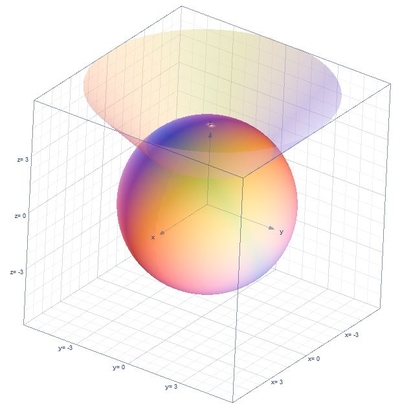# Express the volume of the solid enclosed the cone z=\sqrt{x^{2}+y^{2}} and the sphere...

## Question:

Express the volume of the solid enclosed the cone {eq}\displaystyle z=\sqrt{x^{2}+y^{2}} {/eq} and the sphere {eq}\displaystyle x^{2}+y^{2}+z^{2}=18 {/eq} with triple integral in cylindrical coordinates.

## Volume of the Region:

The triple integrals formula for the volume of the region in cylindrical coordinates is {eq}V=\int_{\alpha }^{\beta }\int_{h_{1}(\theta )}^{h_{2}(\theta )}\int_{u_{1}(r\cos \theta ,r\sin \theta )}^{u_{2}(r\cos \theta ,r\sin \theta )}r\:f\left ( r\cos \theta ,r\sin \theta ,z \right )dzdrd\theta {/eq} and the conversion formulas are {eq}r^{2}=x^{2}+y^{2},\:x=r\cos \theta ,\:y=r\sin \theta {/eq}.

## Answer and Explanation:

Below is the graph,First let us find the limits, substituting the equation {eq}z=\sqrt{x^{2}+y^{2}} {/eq} to the equation {eq}x^{2}+y^{2}+z^{2}=18 {/eq}

{eq}x^{2}+y^{2}+\left ( \sqrt{x^{2}+y^{2}} \right )^{2}=18 {/eq}

{eq}2x^{2}+2y^{2}=18 {/eq}

{eq}x^{2}+y^{2}=9 {/eq}

Thus, the limits are the following

{eq}0\leq \theta \leq 2\pi ,\:0\leq r\leq 3,\:r\leq z\leq \sqrt{18-r^{2}} {/eq}

The volume of the region is ,

{eq}V=\int_{0}^{2\pi }\int_{0}^{3}\int_{r}^{\sqrt{18-r^{2}}}rdzdrd\theta {/eq}

Integrate with respect to {eq}z {/eq}

{eq}V=\int_{0}^{2\pi }\int_{0}^{3}\left [ z \right ]^{\sqrt{18-r^{2}}}_{r}rdrd\theta {/eq}

{eq}V=\int_{0}^{2\pi }\int_{0}^{3}\left ( \sqrt{18-r^{2}}-r \right )rdrd\theta {/eq}

Integrate with respect to {eq}r {/eq}

{eq}V=\int_{0}^{2\pi }\left [ -\frac{1}{3}\left(18-r^2\right)^{\frac{3}{2}}-\frac{r^3}{3} \right ]^{3}_{0}d\theta {/eq}

{eq}V=\int_{0}^{2\pi }\left ( 18\sqrt{2}-18 \right )d\theta {/eq}

Integrate with respect to {eq}\theta {/eq}

{eq}V=\left ( 18\sqrt{2}-18 \right )\left [ \theta \right ]^{2\pi }_{0} {/eq}

{eq}V=2\pi \left(18\sqrt{2}-18\right) {/eq}

{eq}V\approx 46.84645 {/eq}

#### Learn more about this topic:Volumes of Shapes: Definition & Examples

from GMAT Prep: Tutoring Solution

Chapter 11 / Lesson 9
45K